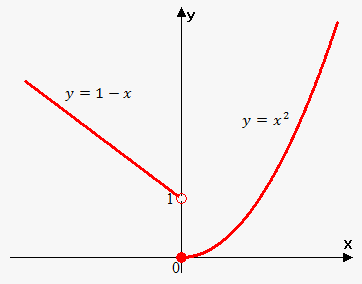# Piecewise Function

When functions is determined by different formulas on different intervals then function is piecewise.

For example, f(x)={(1-x if x<0),(x^2 if x>=0):} is piecewise because on interval (-oo,0) f(x)=1-x and on interval [0,oo) f(x)=x^2.Now find f(-2), f(1), f(0) and draw graph of this function.

Remember that function is a rule. In this case it tells us that if x<0 then apply f(x)=1-x, otherwise apply f(x)=x^2.

Since -2<0 then we apply f(x)=1-x: f(-2)=1-(-2)=3.

Since 1>0 then we apply f(x)=x^2: f(1)=1^2=1.

Since 0>=0 then we apply f(x)=x^2: f(0)=0^2=0.

Now, to draw this function we draw graph of the function f(x)=1-x on interval (-oo,0) and graph of the function f(x)=x^2 on interval [0,oo).

Note, that open dot indicates that it doesn't belong to the graph. Indeed, f(0)=0, so point (0,0) is on the graph, but (0,1) is not.